Following mechanism has been proposed for a reaction,

2A+B $\to$D+E

A+B$\to$ C+D    ...(Slow)

A+ C$\to$ E         ...(Fast)

The rate law expression for the reaction is:

(a) r = K[A]2[B]

(b) r=K[A][B]

(c) r= K[A]2

(d) r= K[A][C]

High Yielding Test Series + Question Bank - NEET 2020

Difficulty Level:

In gaseous reactions important for the understanding of the upper atmosphere H2O and O react bimolecularly to form two OH radicals. $∆$H for this reaction is 72kJ at 500 K and Ea is 77 kJ mol-1, then Ea for the bimolecular recombination of two OH radicals to form H2O and O is:

(a) 3 kJ mol-1

(b) 4 kJ mol-1

(c) 5 kJ mol-1

(d) 7 kJ mol-1

High Yielding Test Series + Question Bank - NEET 2020

Difficulty Level:

For a reaction A Product, rate law is $-\frac{d\left[A\right]}{dt}=K\left[A{\right]}_{0}$. The concentration of A left after time t when $t=\frac{1}{K}$ is:

(a) $\frac{\left[A{\right]}_{0}}{e}$

(b)

(c) $\frac{\left[A{\right]}_{0}}{{e}^{2}}$

(d) $\frac{1}{\left[A{\right]}_{0}}$

High Yielding Test Series + Question Bank - NEET 2020

Difficulty Level:

For an exothermic chemical process occurring in two steps as;

(i) A+B$\to$X(Slow)

(ii) X$\to$AB (Fast)

The progress of the reaction can be best described by:

(a)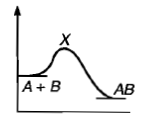(b)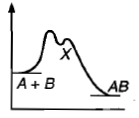(c)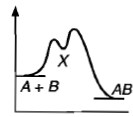(d) all of these

High Yielding Test Series + Question Bank - NEET 2020

Difficulty Level:

For the non-stoichiometric reaction 2A + B $\to$ C +D, the following kinetic data were obtained in three separate experiments, all at 298 K.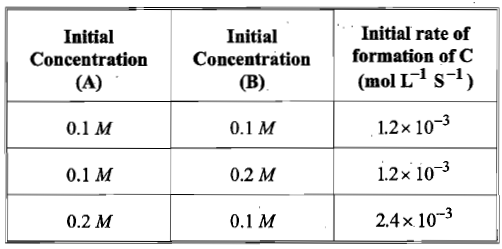The rate law for the formation of C is:

(a) d[C]/dt = k[ A][B]

(b) d[C]/dt = k[ A]2[B]

(c) d[C]/dt = k[ A][B]2

(d) d[C]/dt = k[ A]

High Yielding Test Series + Question Bank - NEET 2020

Difficulty Level:

For the reaction N2 + 3H2 $\to$ 2NH3, the rate d[NH3]/dt = 2x10-4 Ms-1 .Therefore, the rate -d[N2]/dt is given as:

(a) 10-4 Ms-1

(b) 104 Ms-1

(c) 10-2 sM-1

(d) 10-4 sM-1

High Yielding Test Series + Question Bank - NEET 2020

Difficulty Level:

If 'I' is the intensity of absorbed light and 'c' is the concentration of AB for the photochemical process AB + hv→  AB *, the rate of formation of AB * is directly proportional to:

(a) c

(b) I

(c) I2

(d) cI

High Yielding Test Series + Question Bank - NEET 2020

Difficulty Level:

Which curve represents zero order reaction?

(a)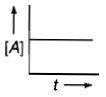(b)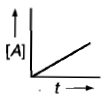(c)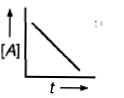(d)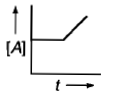High Yielding Test Series + Question Bank - NEET 2020

Difficulty Level:

In a reaction, the rate expression is, rate = K[A][B]2/3[C]0 , the order of reaction is:

1. 1

2. 2

3. 5/3

4. zero

High Yielding Test Series + Question Bank - NEET 2020

Difficulty Level:

The rate of a reaction get doubles when the temperature changes from 7°C to 17°C. By what factor will it change for the temperature change from 17°C to 27°C?

(a) 1.81

(b) 1.71

(c) 1.91

(d) 1.76